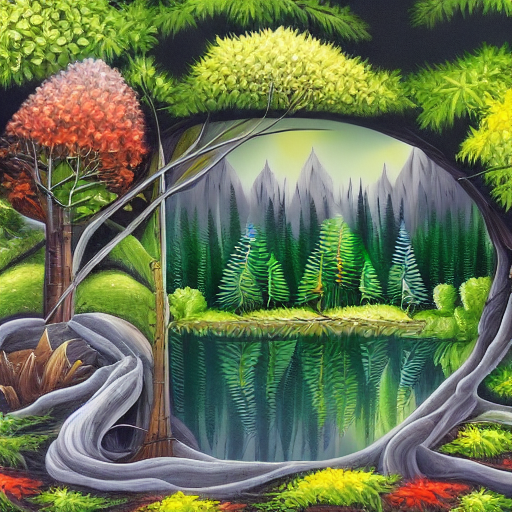David's Blog

# Python script to determine the size of objects in an image

By David Li on Fri, 14 June 2024To build a Python script that detects objects from an input image and outputs the relative size of the objects to the input image, you can use the OpenCV library and the YOLOv3 object detection algorithm. Here’s an example code:

``````import cv2

# Load the YOLOv3 model and its configuration and weights

with open("coco.names", "r") as f:
classes = [line.strip() for line in f.readlines()]

# Get the image dimensions
height, width, channels = image.shape

# Create a blob from the input image and set the input for the YOLOv3 network
blob = cv2.dnn.blobFromImage(image, 1/255, (416, 416), swapRB=True, crop=False)
net.setInput(blob)

# Perform a forward pass through the network to detect objects in the image
outs = net.forward(net.getUnconnectedOutLayersNames())

# Loop through the detected objects and calculate their relative size to the input image
for out in outs:
for detection in out:
scores = detection[5:]
class_id = np.argmax(scores)
confidence = scores[class_id]
if confidence > 0.5:
center_x = int(detection * width)
center_y = int(detection * height)
w = int(detection * width)
h = int(detection * height)
x = int(center_x - w/2)
y = int(center_y - h/2)
cv2.rectangle(image, (x, y), (x + w, y + h), (0, 255, 0), 2)
size = w * h / (width * height)
print("Object: {}, Relative Size: {:.2f}".format(classes[class_id], size))

# Show the output image with object detection and relative size information
cv2.imshow("Output", image)
cv2.waitKey(0)
cv2.destroyAllWindows()``````

In this code, we first load the YOLOv3 model and its configuration and weights, and the class labels for the objects it can detect. We then load the input image and get its dimensions. We create a blob from the image and set it as the input for the YOLOv3 network.

We perform a forward pass through the network to detect objects in the image, and loop through the detected objects. For each object, we calculate its bounding box coordinates and draw a rectangle around it on the input image. We also calculate the relative size of the object to the input image by dividing the area of the bounding box by the area of the input image.

Finally, we display the output image with object detection and relative size information.

© Copyright 2023 by Astro Tech Blog. Built with ♥ by FriendlyUser. Last updated on 2023-09-21.